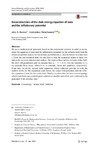## Reconstructions of the dark-energy equation of state and the inflationary potential.

##### Authors
Barrow, John D
Paliathanasis, Andronikoshttps://orcid.org/0000-0002-9966-5517
##### DOIArticle
##### Abstract

We use a mathematical approach based on the constraints systems in order to reconstruct the equation of state and the inflationary potential for the inflaton field from the observed spectral indices for the density perturbations n s and the tensor to scalar ratio r. From the astronomical data, we can observe that the measured values of these two indices lie on a two-dimensional surface. We express these indices in terms of the Hubble slow-roll parameters and we assume that n s - 1 = h r . For the function h r , we consider three cases, where h r is constant, linear and quadratic, respectively. From this, we derive second-order equations whose solutions provide us with the explicit forms for the expansion scale-factor, the scalar-field potential, and the effective equation of state for the scalar field. Finally, we show that for there exist mappings which transform one cosmological solution to another and allow new solutions to be generated from existing ones.

2018
2018-06-19
2018-06-09
##### Keywords
Cosmology, Inflation, Scalar field
##### Journal Title
Gen Relativ Gravit
0001-7701
1572-9532
50
##### Publisher
Springer Science and Business Media LLC